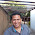## Sunday, January 5, 2020

### Slanted fan-style tree using phytools

Here at the SSB stand-alone meeting (aka. Systematics in the Swamp) in Gainesville, Florida I just saw a talk by Matt McGee in which he showed the following circular (aka. 'fan') slanted tree: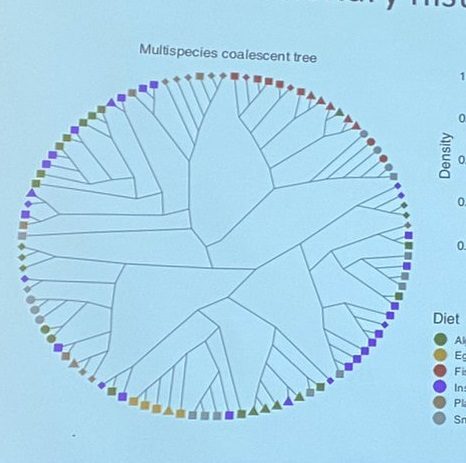So far as I know, there is no option to create such a tree in R. It's not hard to do yourself, however. Let's see how. To do it, I will using `plotTree` and `phylomorphospace`. We will use `plotTree` to find compute the x, y coordinates of the nodes & tips, and then we'll use `phylomorphospace` to draw the lines between them!

``````library(phytools)
tree<-pbtree(n=200) ## simulated tree
## preliminaries
plotTree(tree,fsize=0.5,type="fan",lwd=1,
ftype="off")
``````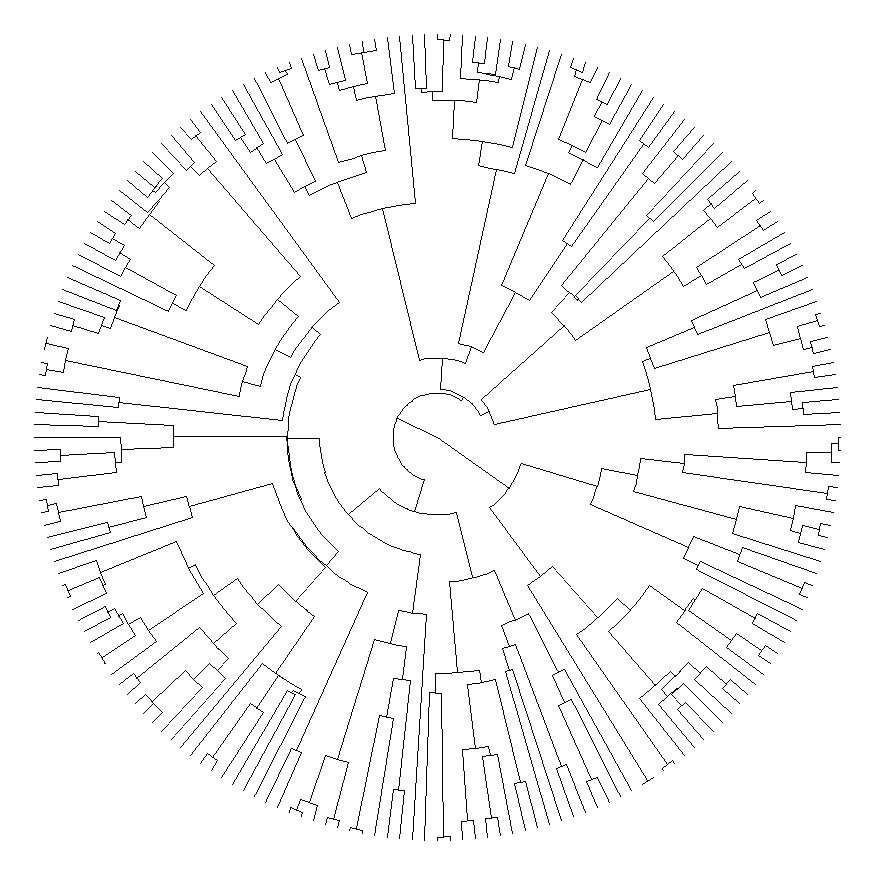``````obj<-get("last_plot.phylo",envir=.PlotPhyloEnv)
X<-cbind(obj\$xx[1:Ntip(tree)],obj\$yy[1:Ntip(tree)])
rownames(X)<-tree\$tip.label
A<-cbind(obj\$xx[1:tree\$Nnode+Ntip(tree)],
obj\$yy[1:tree\$Nnode+Ntip(tree)])
rownames(A)<-1:tree\$Nnode+Ntip(tree)
## now let's create our plot
par(fg="transparent") ## to turn off axes
phylomorphospace(tree,X=X,A=A,ftype="off",
node.size=c(0,0),xlim=obj\$x.lim,
ylim=obj\$y.lim,axes=FALSE)
``````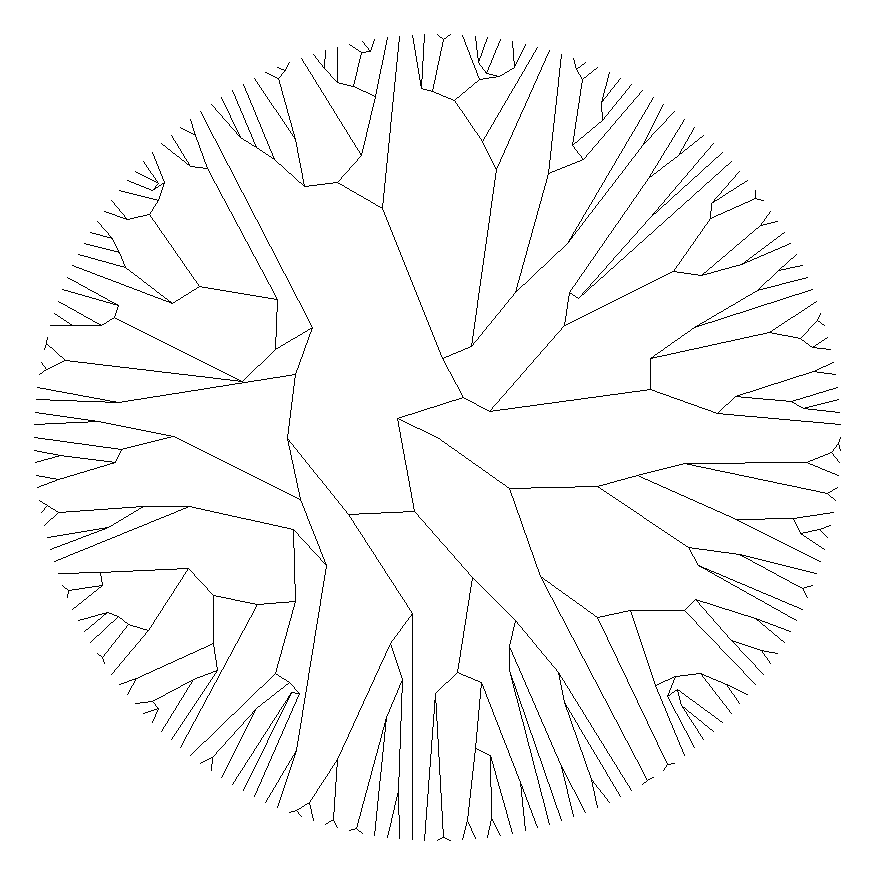``````par(fg="black")
``````

If we'd like, we can also do this showing the topology only, as is often done with slanted trees. Here, I'll use an empirical tree from Near et al. (2011) that can be downloaded here.

``````darter.tree<-read.tree(file="http://www.phytools.org/blog/darter.tre")
plotTree(compute.brlen(darter.tree,power=0.5),
fsize=0.5,type="fan",lwd=1,fsize=0.3,
ftype="i")
``````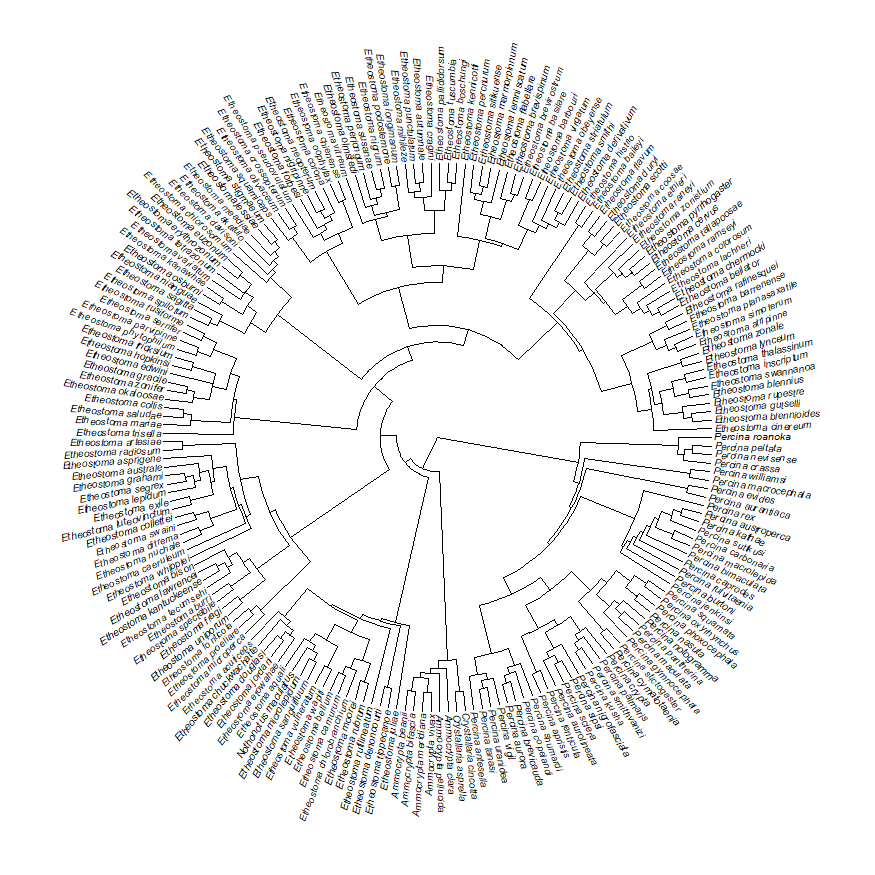``````obj<-get("last_plot.phylo",envir=.PlotPhyloEnv)
X<-cbind(obj\$xx[1:Ntip(darter.tree)],
obj\$yy[1:Ntip(darter.tree)])
rownames(X)<-darter.tree\$tip.label
A<-cbind(obj\$xx[1:darter.tree\$Nnode+
Ntip(darter.tree)],
obj\$yy[1:darter.tree\$Nnode+
Ntip(darter.tree)])
rownames(A)<-1:darter.tree\$Nnode+
Ntip(darter.tree)
par(fg="transparent")
phylomorphospace(darter.tree,X=X,A=A,ftype="off",
node.size=c(0,0),xlim=obj\$x.lim,
ylim=obj\$y.lim,xaxt="n")
par(fg="black")
## as a last step we can put our labels back on
plotTree(darter.tree,fsize=0.5,type="fan",lwd=1,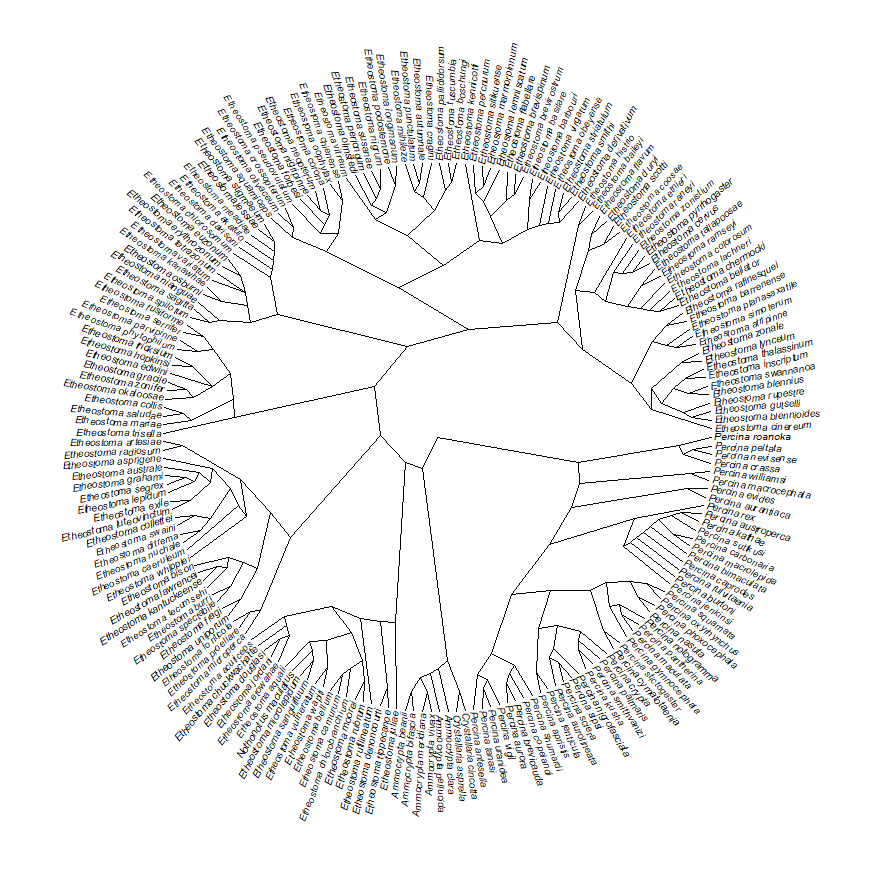1.